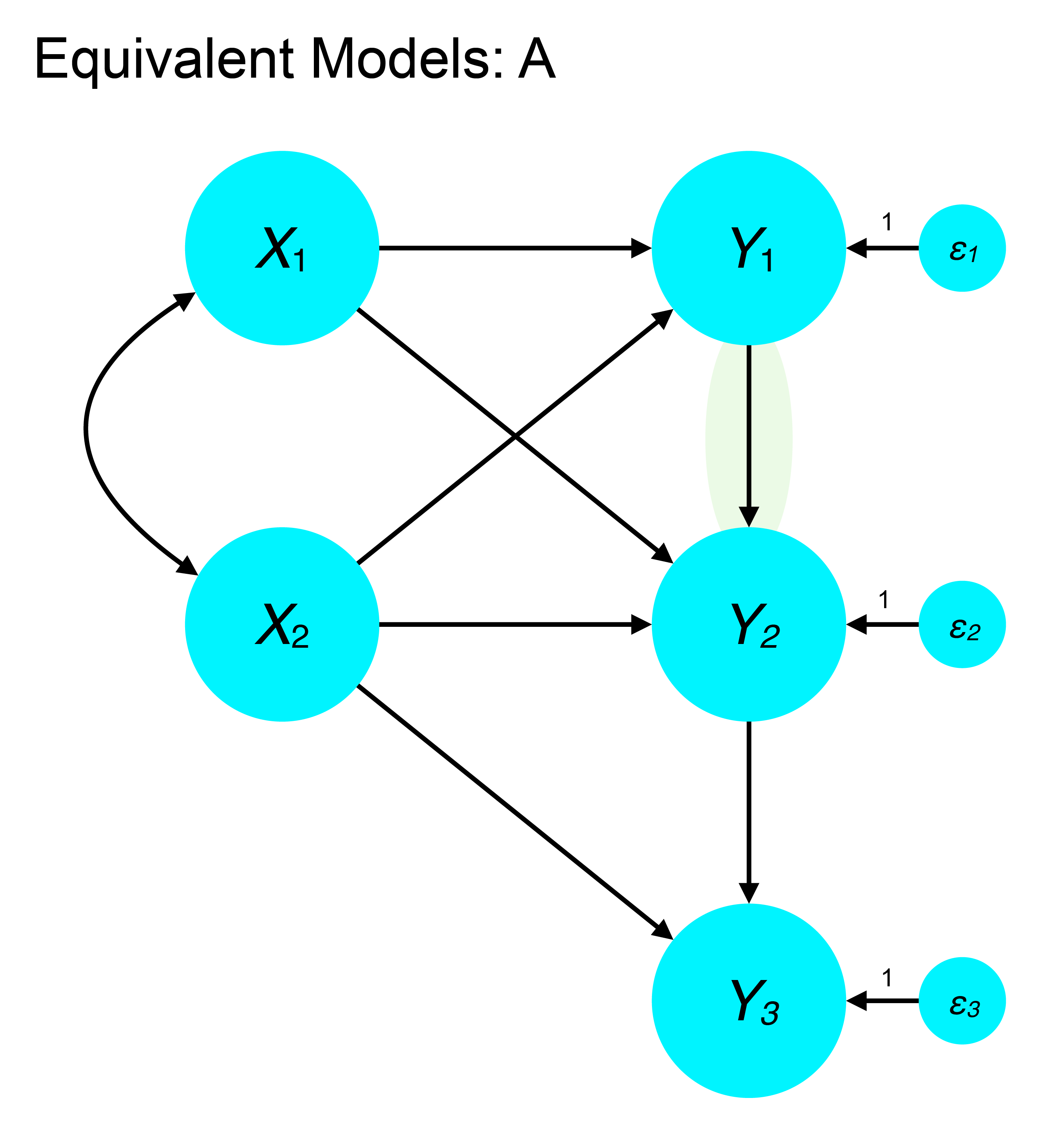# 1 Problems with emphasizing global fit

Tomarken and Waller (2003) note the following problems with models that appear to fit well according to global statistics:

1. There are many equivalent models that fit equally well
2. There may be several alternative models that could fit better
3. Important variables may be omitted (that would affect fit)
4. Fit of lower-order components may be poor
5. An ill-fitting partition (component) may be masked within a well-fitting overall model
6. Global stats may be insensitive to particular kinds of misspecification (esp. in mean structure models)
7. One may make post hoc modifications to the model that reduce the validity and reproducibility of the results.

They also offer the following advice for avoiding overreliance on global fit statistics:

1. Acknowledge plausible equivalent models and design studies to rule out these alternatives
2. Compare the fit of a candidate model against nonequivalent alternatives (e.g., one-factor versus three-factor)
3. Acknowledge potentially important variables that were omitted from the model or not measured. How might these alter the conclusions?
4. Report and evaluate lower-order model components
5. Test components of the model to verify fit in each (this can proceed hierarchically)
6. Design studies with sensitivity to detect non-trivial misspecifications and include measures to flag trivial misspecifications (I think this is quite hard in practice)
7. Clearly distinguish between a priori models and post hoc modifications, or models generated from a search process.

# 2 Equivalent models

Equivalent models have the same degrees of freedom but specify different relationships among variables. Furthermore, equivalent models fit the data equally well, providing identical fit statistics and sample likelihood. These can pose a problem in SEM when our core conclusions rest on specifying the directionality of a relationship if changing the cause and effect would result in equivalent fit.

In general, directed (causal) relations cannot be inferred from covariance data without strong assumptions about the processes that generated the data. For example, if one variable causes another, the cause must precede the effect in time. Likewise, causal relationships are more plausible if one variable is manipulated experimentally and its effects on dependent variables are measured. Thus, the problem of equivalent models largely points to a limitation of relying on observational techniques and correlational measures.

• Observational equivalence: one model generates every probability distribution (univariate or multivariate) generated by an alternative model. In other words, the models cannot be distinguished because the data they generate is identical.
• Covariance equivalence: every covariance matrix possible under one model could be equally generated by another. This leads to the same residual structure and conditional independence relationships.

## 2.1 Lee-Hershberger replacing rules for structural models

• Within a block of variables at the beginning of a structural model that is just identified and with unidirectional relations to subsequent variables, direct effects, correlated disturbances, and equality-constrained reciprocal effects are interchangeable. This means we can replace $$Y1 \rightarrow Y2$$ with $$Y1 \leftarrow Y2$$, $$Y2 \leftrightharpoons Y1$$, or $$D1 \leftrightarrow D2$$.

• At subsequent places in the model where two endogenous variables have the same causes and their relations are unidirectional, all of the following may be substituted for one another: $$Y1 \rightarrow Y2$$ with $$Y1 \leftarrow Y2$$, $$Y2 \leftrightharpoons Y1$$ (equality-constrained), or $$D1 \leftrightarrow D2$$.

Limitations of replacing rules:

1. They may not be transitive: reapplying replacing rules from previous applications may not generate additional versions of the model that are all equivalent.
2. The replacing rules can generate a new structural model whose conditional independence relationships are not identical (a problem with d-separation, as we’ll discuss vis-a-vis causality).

## 2.2 Examples of equivalent models

From Mulaik (2009)

### 2.2.1$$Y1$$ causes $$Y2$$